Home Practice
For learners and parents For teachers and schools
Textbooks
Full catalogue
Pricing SupportLog in

We think you are located in United States. Is this correct?

# 7.2 Triangles

## 7.2 Triangles (EMA5R)

### Classification of triangles (EMA5S)

A triangle is a three-sided polygon. Triangles can be classified according to sides: equilateral, isosceles and scalene. Triangles can also be classified according to angles: acute-angled, obtuse-angled and right-angled.

We use the notation $$\triangle ABC$$ to refer to a triangle with vertices labelled $$A$$, $$B$$ and $$C$$.

 Name Diagram Properties Scalene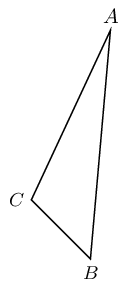All sides and angles are different. Isosceles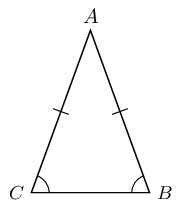Two sides are equal in length. The angles opposite the equal sides are also equal. Equilateral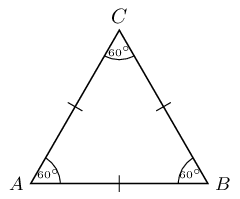All three sides are equal in length and all three angles are equal. Acute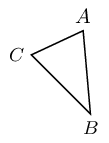Each of the three interior angles is less than $$90°$$. Obtuse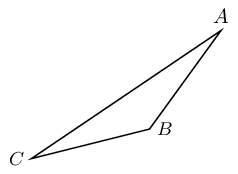One interior angle is greater than $$90°$$. Right-angled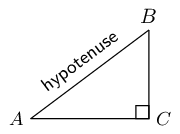One interior angle is $$90°$$.

Different combinations of these properties are also possible. For example, an obtuse isosceles triangle and a right-angled isosceles triangle are shown below: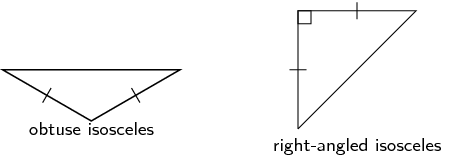This video shows the different ways to classify triangles.

Video: 2G69

## Interior angles of a triangle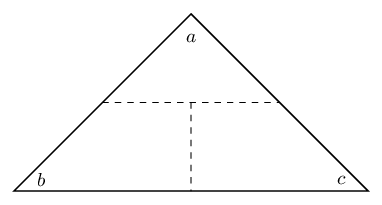1. On a piece of paper draw a triangle of any size and shape.

2. Cut it out and label the angles $$\hat{a}$$, $$\hat{b}$$ and $$\hat{c}$$ on both sides of the paper.

3. Draw dotted lines as shown and cut along these lines to get three pieces of paper.

4. Place them along your ruler as shown in the figure below.

5. What can we conclude?

Hint: What is the sum of angles on a straight line?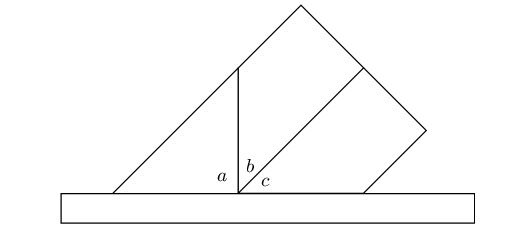## Exterior angles of a triangle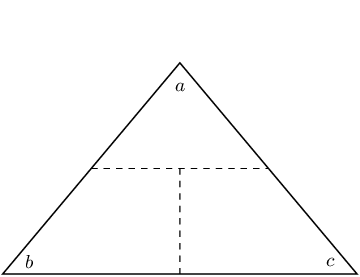1. On a piece of paper draw a triangle of any size and shape. On another piece of paper, make a copy of the triangle.

2. Cut both out and label the angles of both triangles $$\hat{a}$$, $$\hat{b}$$ and $$\hat{c}$$ on both sides of the paper.

3. Draw dotted lines on one triangle as shown and cut along the lines.

4. Place the second triangle and the cut out pieces as shown in the figure below.

5. What can we can conclude?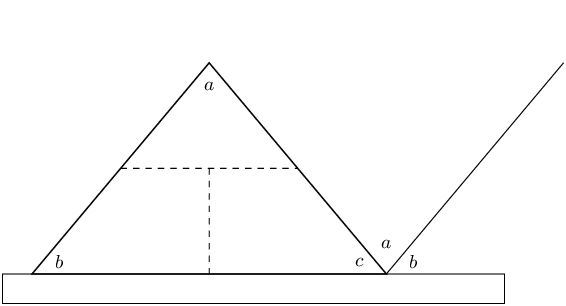We can use the fact that the angles in a triangle add up to $$180^{\circ}$$ to work out the sum of the exterior angles in a pentagon. This video shows you how.

Video: 2G6B

### Congruency (EMA5T)

Two triangles are congruent if one fits exactly over the other. This means that the triangles have equal corresponding angles and sides. To determine whether two triangles are congruent, it is not necessary to check every side and every angle. We indicate congruency using $$\equiv$$.

The following table describes the requirements for congruency:

 Rule Description Diagram RHS or $$90°$$HS ($$90°$$, hypotenuse, side) If the hypotenuse and one side of a right-angled triangle are equal to the hypotenuse and the corresponding side of another right-angled triangle, then the two triangles are congruent.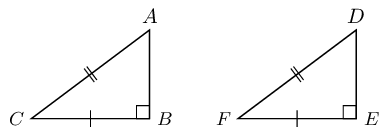$$\triangle ABC\equiv \triangle DEF$$ SSS (side, side, side) If three sides of a triangle are equal in length to the corresponding sides of another triangle, then the two triangles are congruent.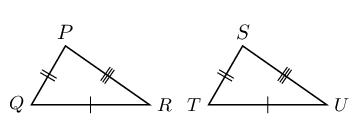$$\triangle PQR\equiv \triangle STU$$ SAS or S$$\angle$$S (side, angle, side) If two sides and the included angle of a triangle are equal to the corresponding two sides and included angle of another triangle, then the two triangles are congruent.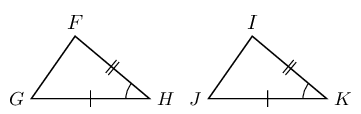$$\triangle FGH\equiv \triangle IJK$$ AAS or $$\angle \angle$$S (angle, angle, side) If one side and two angles of a triangle are equal to the corresponding one side and two angles of another triangle, then the two triangles are congruent.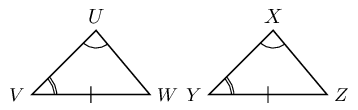$$\triangle UVW\equiv \triangle XYZ$$

The order of letters when labelling congruent triangles is very important.

$\triangle ABC\equiv \triangle DEF$

This notation indicates the following properties of the two triangles: $$\hat{A}=\hat{D}$$, $$\hat{B}=\hat{E}$$, $$\hat{C}=\hat{F}$$, $$AB=DE$$, $$AC=DF$$ and $$BC=EF$$.

You might see $$\cong$$ used to show that two triangles are congruent. This is the internationally recognised symbol for congruency.

This video shows some practice examples of finding congruent triangles.

Video: 2G6C

temp text

### Similarity (EMA5V)

Two triangles are similar if one triangle is a scaled version of the other. This means that their corresponding angles are equal in measure and the ratio of their corresponding sides are in proportion. The two triangles have the same shape, but different scales. Congruent triangles are similar triangles, but not all similar triangles are congruent. We use $$\enspace|||\enspace$$ to indicate that two triangles are similar.

The following table describes the requirements for similarity:

 Rule Description Diagram AAA (angle, angle, angle) If all three pairs of corresponding angles of two triangles are equal, then the triangles are similar.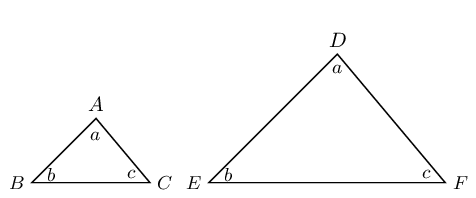$$\hat{A} = \hat{D}$$, $$\hat{B} = \hat{E}$$, $$\hat{C} = \hat{F}$$ $$\therefore \triangle ABC \enspace ||| \enspace \triangle DEF$$ SSS (side, side, side) If all three pairs of corresponding sides of two triangles are in proportion, then the triangles are similar.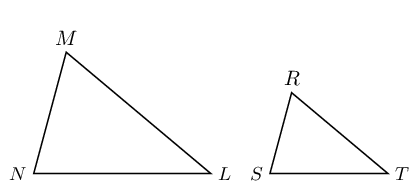$$\frac{MN}{RS}=\frac{ML}{RT}=\frac{NL}{ST}$$ $$\therefore \triangle MNL \enspace|||\enspace \triangle RST$$

The order of letters for similar triangles is very important. Always label similar triangles in corresponding order. For example,

\begin{align*} \triangle MNL \enspace|||\enspace \triangle RST &\text{ is correct; but } \\ \triangle MNL \enspace|||\enspace \triangle RTS & \text{ is incorrect.} \end{align*}

You might see $$\sim$$ used to show that two triangles are similar. This is the internationally recognised symbol for similarity.

The following video explains similar triangles.

Video: 2G6D

temp text

### The theorem of Pythagoras (EMA5W)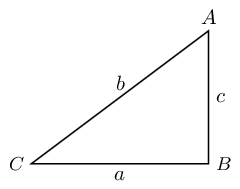If $$\triangle ABC$$ is right-angled with $$\hat{B}= 90°$$, then $${b}^{2} = {a}^{2} + {c}^{2}$$.

Converse: If $${b}^{2} = {a}^{2} + {c}^{2}$$, then $$\triangle ABC$$ is right-angled with $$\hat{B} = 90°$$.

The following video explains the theorem of Pythagoras and shows some examples of working with the theorem of Pythagoras.

Video: 2G6F

## Worked example 2: Triangles

Determine if the two triangles are congruent. Use the result to find $$x$$, $$\hat{y}$$ and $$z$$.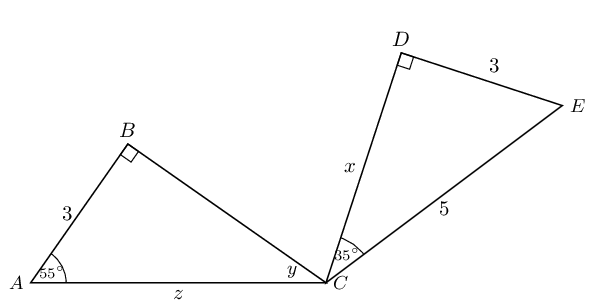### Determine whether $$\triangle CDE\equiv \triangle CBA$$

In $$\triangle CDE$$:

\begin{align*} \hat{D} + \hat{C} + \hat{E} & = 180° \qquad \text{(sum of }\angle \text{s} \text{ in }\triangle \text{)}\\ 90° + 35° + \hat{E} & = 180° \\ \therefore \hat{E} & = 55° \end{align*}

In $$\triangle CDE$$ and $$\triangle CBA$$:

$\begin{array}{rll} D\hat{E}C & = B\hat{A}C = 55° & \text{(proved)} \\ C\hat{D}E & = C\hat{B}A = 90° & \text{(given)}\\ DE & = BA = 3 & \text{(given)} \\ \therefore \triangle CDE& \equiv \triangle CBA & \text{(AAS)} \end{array}$

### Determine the unknown angles and sides

In $$\triangle CDE$$:

\begin{align*} C{E}^{2} & = D{E}^{2} + C{D}^{2} \qquad \text{(Pythagoras)} \\ {5}^{2} & = {3}^{2} + {x}^{2} \\ {x}^{2} & = 16 \\ \therefore x & = 4 \end{align*}

In $$\triangle CBA$$:

\begin{align*} \hat{B} + \hat{A} + \hat{y} & = 180° \qquad \text{(sum of } \angle \text{s in }\triangle \text{)}\\ 90° + 55° + \hat{y} & = 180° \\ \therefore \hat{y} & = 35° \end{align*}\begin{align*} \triangle CDE& \equiv \triangle CBA \qquad \text{(proved)}\\ \therefore CE & = CA \\ \therefore z & = 5 \end{align*}
temp text
Textbook Exercise 7.2

Calculate the unknown variables in each of the following figures.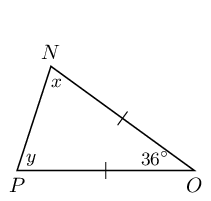The triangle is isosceles therefore $$x = y$$ ($$\angle$$s opp equal sides).

\begin{align*} 180° & = 36° - 2x \qquad \text{(sum of }\angle \text{s} \text{ in }\triangle \text{)} \\ 2x & = 144° \\ \therefore x & = 72° = y \end{align*}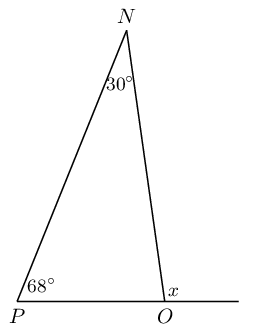$$x$$ is an exterior angle, therefore $$P\hat{N}O + O\hat{P}N = x$$ (ext $$\angle$$ of $$\triangle$$).

\begin{align*} x & = 30° + 68° \\ &= 98° \end{align*}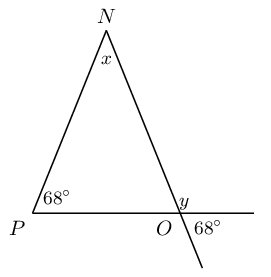First find $$y$$. $$y + 68° = 180°$$ ($$\angle$$s on a str line). Therefore $$y = 112°$$.

$$y$$ is an exterior angle, therefore $$P\hat{N}O + O\hat{P}N = y$$ (ext $$\angle$$ of $$\triangle$$).

\begin{align*} 112° & = x + 68°\\ x & = 112° - 68° \\ & = 44° \end{align*}

Therefore $$y = 112°$$ and $$x = 44°$$.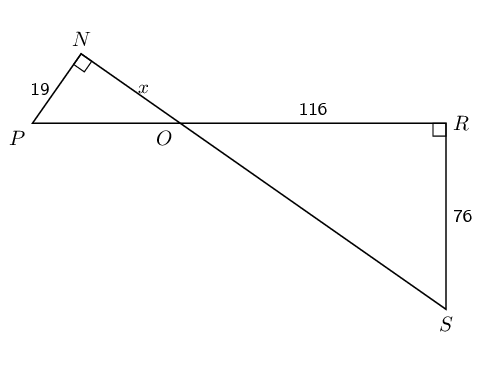\begin{align*} N\hat{P}O & = 180° - P\hat{N}O - N\hat{O}P \qquad \text{(sum of } \angle \text{s in } \triangle \text{)}\\ & = 180° - 90° - N\hat{O}P \\ & = 90° - N\hat{O}P \end{align*} \begin{align*} R\hat{S}O & = 180° - O\hat{R}S - R\hat{O}S \qquad \text{(sum of } \angle \text{s in } \triangle \text{)}\\ & = 180° - 90° - R\hat{O}S \\ & = 90° - R\hat{O}S \end{align*}

$$N\hat{O}P = R\hat{O}S$$ (vert opp $$\angle$$s).

$$\therefore N\hat{P}O = R\hat{S}O$$.

Therefore $$\triangle NPO$$ and $$\triangle ROS$$ are similar because they have the same angles.

Similar triangles have proportional sides:

\begin{align*} \therefore \frac{NP}{RS} &= \frac{NO}{OR}\\ \frac{19}{76}&=\frac{x}{116}\\ \therefore x &= 29 \end{align*}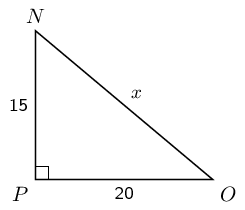From the theorem of Pythagoras we have:

\begin{align*} x^2 &= 15^2 + 20^2 \\ \therefore x &= \sqrt{625} \\ &= 25 \end{align*}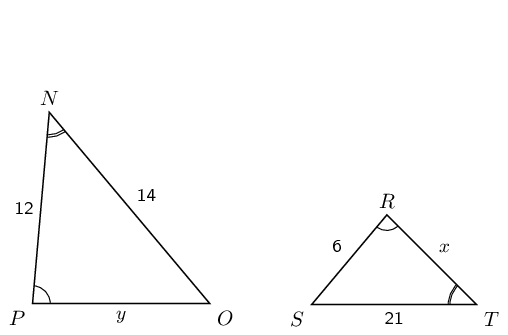We note that:

\begin{align*} N\hat{P}O & = S\hat{R}T \qquad \text{(given)} \\ P\hat{N}O & = R\hat{T}S \qquad \text{(given)} \\ \therefore P\hat{N}O & = R\hat{T}S \qquad \text{(sum of} \angle \text{s in } \triangle \text{)} \\ \therefore \triangle NPO &\enspace|||\enspace \triangle TSR \text{ (AAA)} \end{align*}

Now we can use the fact that the sides are in proportion to find $$x$$ and $$y$$:

\begin{align*} \frac{NO}{OP} &= \frac{TS}{TR} \\ \frac{14}{12} &= \frac{21}{x} \\ x &= \frac{21 \times 12}{14} \\ &= 18 \\\\ \frac{OP}{NP} &= \frac{SR}{TR}\\ \frac{y}{12} & = \frac{6}{18} \\ 18y & = 72 \\ y & = 4 \end{align*}

Therefore $$x = 18$$ and $$y = 4$$.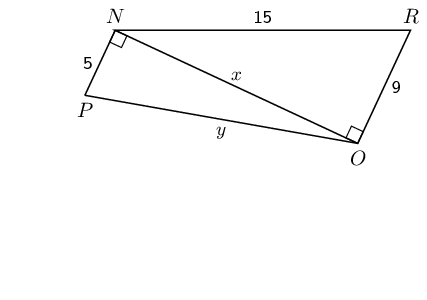From the theorem of Pythagoras:

\begin{align*} x^2 & = 15^2 - 9^2\\ x & = \sqrt{144}\\ & = 12 \\ \\ y^2 & = x^2 + 5^2 \\ y^2 & = 144 + 25 \\ y & = \sqrt{169} \\ y & = 13 \end{align*}

Therefore $$x = 12$$ and $$y = 13$$.

Given the following diagrams:

Diagram A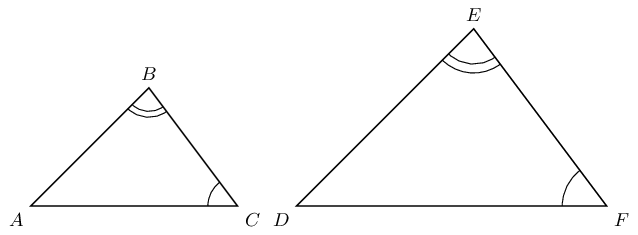Diagram B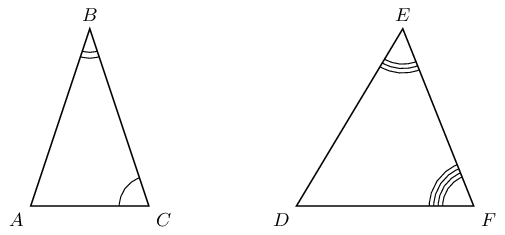Which diagram correctly gives a pair of similar triangles?

Diagram A shows a pair of triangles with all pairs of corresponding angles equal (the same three angle markers are shown in both triangles). Diagram B shows a pair of triangles with different angles in each triangle. All six angles are different and there are no pairs of corresponding angles that are equal.

Therefore diagram A gives a pair of triangles that are similar.

Given the following diagrams:

Diagram A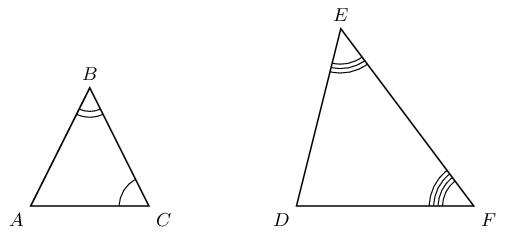Diagram B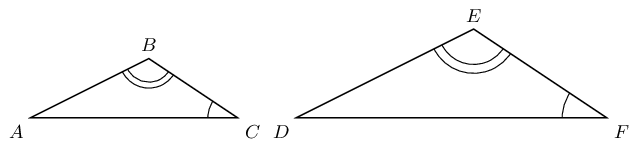Which diagram correctly gives a pair of similar triangles?

Diagram A shows a pair of triangles with different angles in each triangle. All six angles are different and there are no pairs of corresponding angles that are equal. Diagram B shows a pair of triangles with all pairs of corresponding angles equal (the same two angle markers are shown in both triangles and the third angle in each triangle must be equal).

Therefore diagram B gives a pair of triangles that are similar.

Have a look at the following triangles, which are drawn to scale: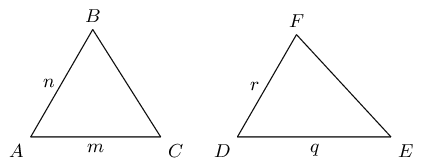Are the two triangles congruent? If so state the reason and use the correct notation to state that they are congruent.

We are not told if $$n = r$$ and $$m = q$$ or $$n = q$$ and $$m = r$$ therefore we cannot say that the sides are the same length. Also we are not given any information about the angles of the two triangles. Therefore we cannot say if the two triangles are congruent.

Have a look at the following triangles, which are drawn to scale: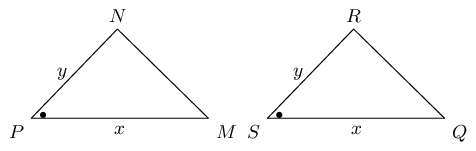Are the two triangles congruent? If so state the reason and use the correct notation to state that they are congruent.

Note that the two pairs of sides are equal, as indicated by the $$x$$ and $$y$$. In addition, the angle between those two sides are marked as equal (this is the included angle).

Therefore, these two triangles are congruent. $$\triangle PNM \equiv \triangle QSR$$, reason: SAS.

State whether the following pairs of triangles are congruent or not. Give reasons for your answers. If there is not enough information to make a decision, explain why.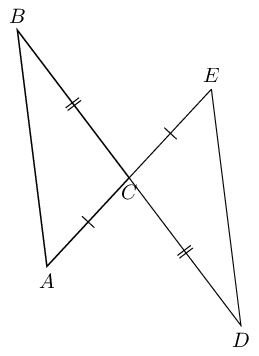\begin{align*} AC & = CE \qquad \text{(given)} \\ BC & = CD \qquad \text{(given)} \\ A\hat{C}B & = D\hat{C}E \qquad \text{(vert opp } \angle \text{s} = \text{)} \\ \therefore \triangle ABC & \equiv \triangle EDC \qquad \text{SAS} \end{align*}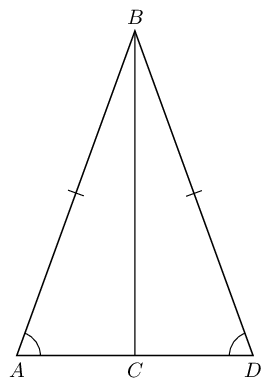We have two equal sides ($$AB = BD$$ and $$BC$$ is common to both triangles) and one equal angle ($$\hat{A} = \hat{D}$$) but the sides do not include the known angle. The triangles therefore do not have a SAS and are therefore not congruent. (Note: $$A\hat{C}B$$ is not necessarily equal to $$D\hat{C}B$$ because it is not given that $$BC \perp AD$$).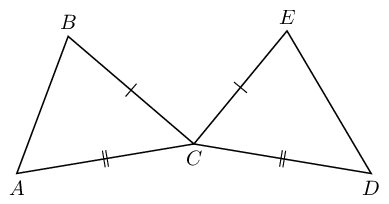There is not enough information given. We need at least three facts about the triangles and in this example we only know two sides in each triangle.

Note that $$BCD$$ and $$ECA$$ are not straight lines and so we cannot use vertically opposite angles.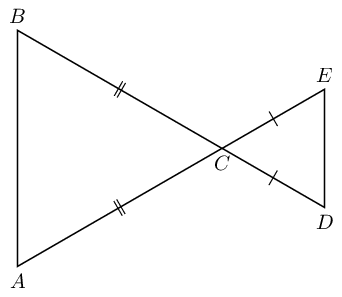There is not enough information given. Although we can work out which angles are equal we are not given any sides as equal. All we know is that we have two isosceles triangles. Note how this question differs from part a). In part a) we were given equal sides in both triangles, in this question we are only given that sides in the same triangle are equal.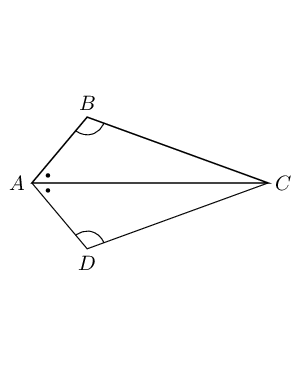\begin{align*} AC & = AC \qquad \text{(common side)} \\ B\hat{A}C & = D\hat{A}C \qquad \text{(given)} \\ A\hat{B}C & = A\hat{D}C \qquad \text{(given)} \\ \therefore \triangle ABC & \equiv \triangle ADC \qquad \text{AAS} \end{align*}